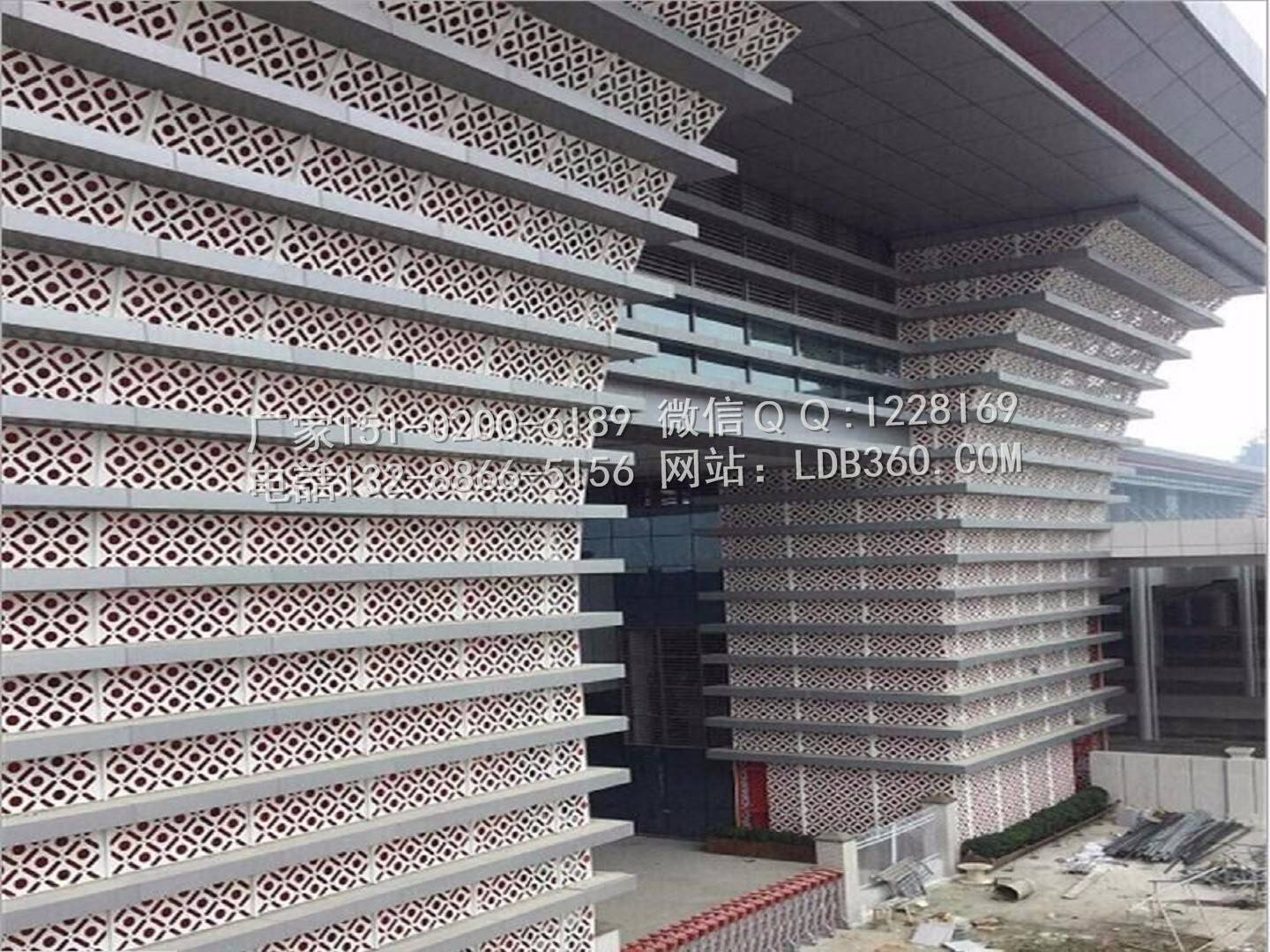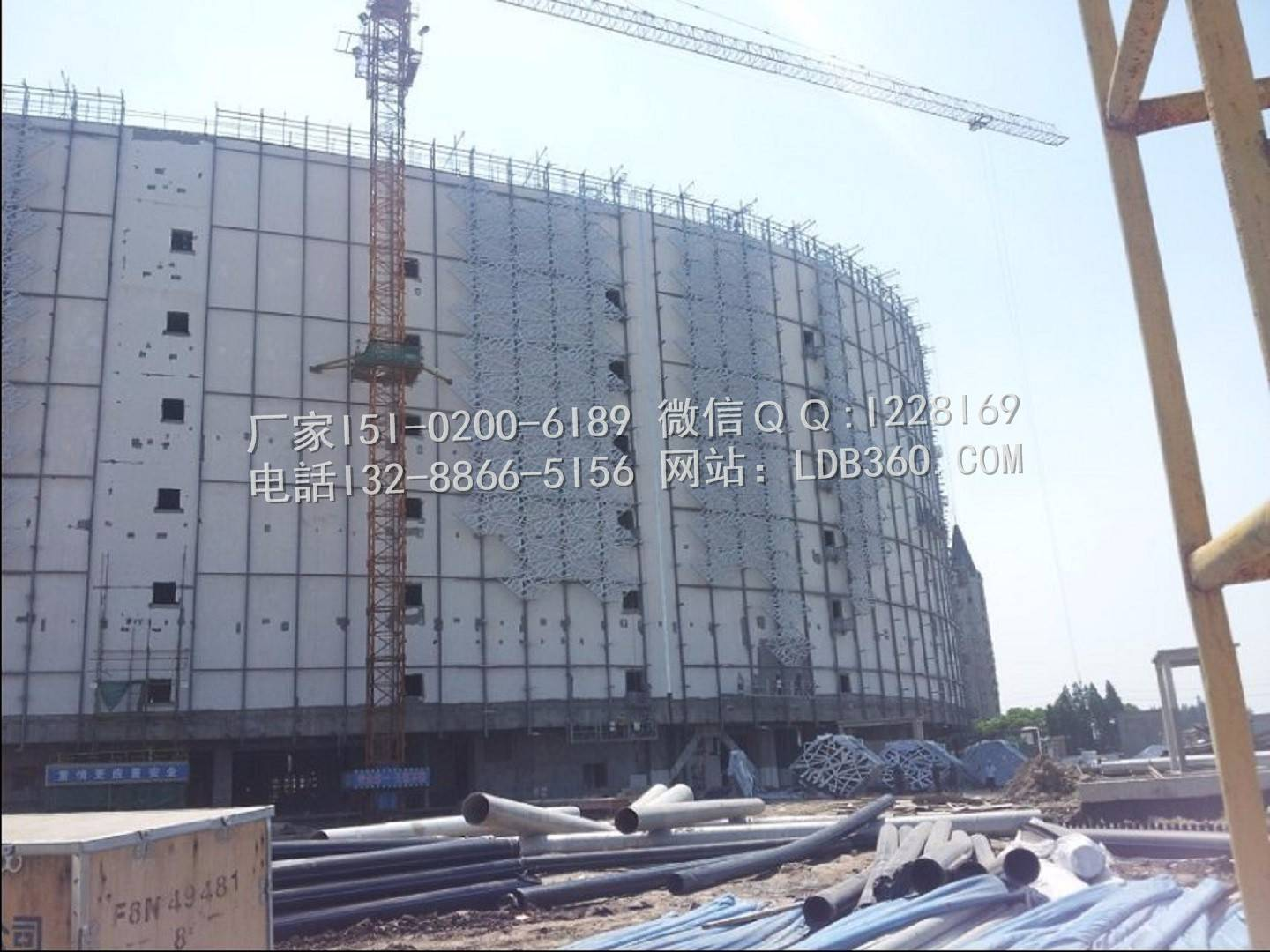# 《金属幕墙工程技术规范》铝板强度及挠度计算！

2018/10/10 宏铝建材

《金属幕墙工程技术规范》铝板强度及挠度计算！

计算条件：铝板宽度                           A=1200mm

劲筋间距                           B=600mm

铝板厚度                           T=3mm

计算模型为四边简支板。

根据您输入的数据，

风荷载标准值                     Wk =-1.2 kN/m^2

根据您输入的数据，       抗震烈度7度(0.15g)

分布水平地震作用标准值     qEk=0.2 kN/m^2依据：《金属幕墙工程技术规范》JGJ133-2001计算公式。

风荷载的分项系数取1.4，地震作用的分项系数取1.3.

风荷载的组合系数取1，地震作用的组合系数取0.5.

q =1.4*Wk+0.5*1.3*qEk

=1.4 *-1.2+0.5*1.3*0.2

=1.836 kN/m^2校核依据：δ=6*m*q*a^2*η/t^2[fg]=81kN/m^2

可查《金属与石材幕墙工程技术规范》JGJ133-2001,附录B 板弯矩系数B.0.1

铝板计算弯矩系数

m=0.1022

根据《金属与石材幕墙工程技术规范》JGJ133-2001,5.4.3第四条公式5.4.3-3

系数:

θ=q*a ^ 4 / (E * t ^ 4)

=1.836*600^ 4 / (0.7* 10 ^ 5 *3^ 4)

=41965.71

根据《金属与石材幕墙工程技术规范》JGJ133-20015.4.31条：

当板的挠度大于板的厚度时，应本条第四款的规定考虑大挠度的影响，即将应力值乘以应折减系统。

根据θ，查《金属与石材幕墙工程技术规范》JGJ133-20015.4.3可得。得应力折减系数η。

应力折减系数:            η=0.4

铝板厚度:                    t=3 mm

铝板的短边边长:         a=600 mm

在风荷载和垂直于板面方向地震作用在板中产生的最大弯曲应力设计值:

δ=6*m*q*a^2*η/t^2

=6*0.1022*1.836*600^ 2/3^ 2/ 1000

=45.03kN/m^2<81 kN/m^2校核依据：f=μ*Wk*a^4*η/D [μ]=a/100=6mm

风荷载标准值：

Wk =-1.2 kN/m^2

查《金属与石材幕墙工程技术规范》JGJ133-20015.4.3可得:

挠度系数:

μ=0.00488

根据铝板相关输入参数，可以计算:

铝板刚度:

D=70000* t^ 3 / (12 * (1 - 0.33 ^ 2))

=70000 *3^ 3 / (12 * (1 - 0.33 ^ 2))

=176747.84

根据下面的系数:

系数:

θ=Wk * b0^ 4 / (7 * 10 ^ 4 * t^ 4 * 1000)

=1.2*600^ 4 / (7 * 10 ^ 4 *3^ 4 * 1000)

=27.43

根据《金属与石材幕墙工程技术规范》JGJ133-20015.4.35条：

当进行板的挠度计算时，也应考虑大挠度的影响，按小挠度公式计算的挠度值也应乘以折减系数。

折减系数:

η=0.84

铝板挠度：

f=μ* Wk * b0 ^ 4 * η / ( D * 1000)

=0.00488*1.2*600^ 4 *0.84343/ (176747.84* 1000)

=3.62mm<6mm铝板强度及挠度符合规范要求！